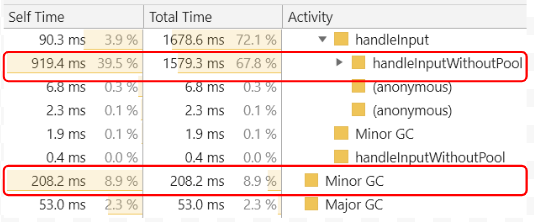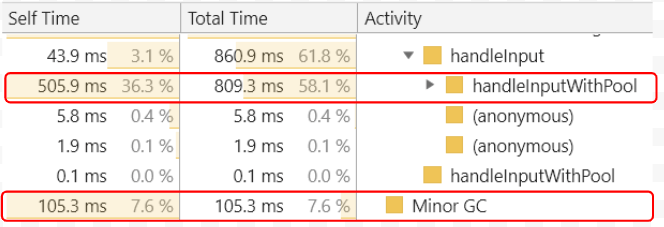# Object Pool use case

After the Object Pool article went online, I was asked this on Facebook:Benjamin Gruenbaum comment on my article. Benji is a nodejs contributor, among other cool things he’s doing.

What I take from here is 2 fold:

1. The one paragraph spent explaining about use cases might not be enough – we need an example that is somewhat like real life.
2. From the article, it might seem that Object Pool is the way to handle every array you create (actually, I got this remark from someone who came to talk to me after one of my talks).

I’ve spent some time building a scenario similar to one of the app’s I’ve built.

In this app, when the app loaded, the server sent a message with anything between 1000 to 50000 entities.

From then on, every 200 milliseconds or so, an update is pushed to the client. This update would be a bulk update that could convey:

1. Create entity
2. Delete entity
3. Update entity

For the purpose of this demo, I conduct only Create and Delete*. In addition, instead of a server, I’m using a web worker to push data.

* In my case, the Update operation was also causing GC, because it was updating nested objects (e.g. replacing `a` in `x = { a: {//...} }`.

Each example simulates 200 messages from the server.

The results for the non object pool example are shown in Figure 1.

Figure 1 indicates that 220ms were spent on Minor GC and 53ms were spent on Major GC during the run of these 200 messages.

Figure 2 shows what happens when we are running the same scenario with an object pool.

The data in Figure 2 shows that using object pool the Minor GC time was reduced by half. Moreover, the time spent on Major GC was reduced much more (almost 90%).

### Memory allocation and total runtime

A lot of GC usually comes with a heavy memory allocation process. We can see that the GC cost us arount 150ms in the example above. How much is it from the total difference?

Figure 3 and Figure 4 summarize the main functions and GC in one table for both the non Object Pool and the Object Pool case respectively.

In Figure 3 we see the data handling took 1579ms while in Figure 4 we see that the data handling took 809ms. That’s almost half!Figure 3: Top red rectangle – the amount of time it took the “Naive” algorithm to handle 200 incoming updates: 809.3ms. Bottom red rectangle – the amount of time spent in minor GC.Figure 4: Top red rectangle – the amount of time it took Object Pool powered algorithm to handle 200 incoming updates: 809.3ms. Bottom red rectangle – the amount of time spent in minor GC.

Of course, the longer the app runs (SPA anyone) and more messages are received from the server, the greater the difference between the two.

## The GOTCHA

I’d like to emphasize the importance of monitoring.

In the case presented here, the `Demo` class has around 150 properties. It can be 150 properties or multiple nested properties.

It might be, though, that in your app the class you instantiate is very simple.

This is why the demo allows you to play both with the class size as well as the amount of iterations.

When monitoring the app with a smaller `Demo` class (e.g. less properties), you’d might see that without an object pool you will get better performance.Figure 5: The results of a test with an empty Demo class (number of properties 0). The Object Pool function took about the same time as the function without the pool.

This should give you pause and think – when should I use an object pool (or any design pattern)?

The answer is – monitor. Every design pattern has a problem it’s supposed to solve.

In the object pool case, check how much GC you get in your app. Then, if the GC is generated mostly by you instantiating and deleting similar objects – it might be that an Object Pool is the right way to go.

## Summary

Thanks to the comment by Benjamin Gruenbaum, I’ve taken the time to sit down and recreate a situation I ran into in production.

I hope this example emphasizes the importance of understanding what’s happening in your application in regards to memory consumption.

Remember to always monitor. Take into account that the code here is very simple. Your usual app will probably have more complex objects as input. The bigger the objects, the more impact their Create and Delete operations will have.

On the other hand, if they are very simple – allocation and GC might not be your problem…

Remember you can monitor the example yourself here: https://yonatankra.com/performance/memory/demos/realLifeObjectPool/

The code is here:

 (function () { class PoolObject { constructor(data) { this.data = data; this.nextFree = null; this.previousFree = null; this.free = true; } } class Pool { constructor(objCreator, objReseter, initialSize = 5000) { this._pool = []; this.objCreator = objCreator; this.objReseter = objReseter; for (let i = 0; i < initialSize; i++) { this.addNewObject(this.newPoolObject()); } } addNewObject(obj) { this._pool.push(obj); this.release(obj); return obj; } release(poolObject) { // flag as free poolObject.free = true; // set in the dequeue poolObject.nextFree = null; poolObject.previousFree = this.lastFree; // if we had a last free, set the last free's next as the new poolObject // otherwise, this is the first free! if (poolObject.previousFree) { this.lastFree.nextFree = poolObject; } else { this.nextFree = poolObject; } // set the new object as the last in the dequeue this.lastFree = poolObject; // reset the object if needed this.objReseter(poolObject); } getFree() { // if we have a free one, get it - otherwise create it // if (!this.nextFree) { // for (let i = 0; i < this._pool.length / 2; i++) { // this.addNewObject(this.newPoolObject()); // } // } const freeObject = this.nextFree ? this.nextFree : this.addNewObject(this.newPoolObject()); // flag as used freeObject.free = false; // the next free is the object's next free this.nextFree = freeObject.nextFree; // if there's nothing afterwards, the lastFree is null as well if (!this.nextFree) this.lastFree = null; // return the now not free object return freeObject; } newPoolObject() { const data = this.objCreator(); return new PoolObject(data, this.lastFree, this.nextFree); } releaseAll() { this._pool.forEach(item => this.release(item)); } } class Demo { constructor(someVariable = null) { this.counter = someVariable; for (let i = 0; i < 150; i++) { this[i] = i; } } demoMethod(val) { return val % 2; } demoMethod2(val) { return val * 2; } demoMethod3(val) { return val + 2; } demoMethod4(val) { return val - 2; } } const MAX_MESSAGES = 200; const counters = { deletions: 0, additions: 0 }; const mockServer = new Worker("worker.js"); mockServer.onmessage = function (e) { // console.debug('Data received from server'); const input = e.data; handleInput(input); }; let pool; let currState; let dataSet = {}; let inputHandling = 0; function handleInput(input) { if (currState === 'Pool') { handleInputWithPool(input); } else if (currState === 'NoPool') { handleInputWithoutPool(input); } inputHandling++; if (inputHandling === 1) { // console.log('Finished filling up the dataSet for the first time'); return; } counters.deletions += input.reduce((a,b) => a + (b.action ? 0 : 1), 0); counters.additions += input.reduce((a,b) => a + (b.action ? 1 : 0), 0); if (inputHandling === MAX_MESSAGES) { console.log(`Totals: \${JSON.stringify(counters)}`); } } function handleInputWithoutPool(input) { for (let i = 0; i < input.length; i++) { const id = input[i].payload.id; switch (input[i].action) { case 0: // delete delete dataSet[id]; break; case 1: // create dataSet[id] = new Demo(id); break; case 2: // TODO::update break; } } } function handleInputWithPool(input) { let object; for (let i = 0; i < input.length; i++) { const id = input[i].payload.id; switch (input[i].action) { case 0: // delete object = dataSet[id]; delete dataSet[id]; if (object) { pool.release(object); //TODO::expose the pool globally } break; case 1: object = pool.getFree(); object.data.counter = i; dataSet[id] = object; break; case 2: // TODO::update break; } } } function createAndDestroy() { currState = 'NoPool'; generalReset(); } function createWithAPool() { currState = 'Pool'; pool = new Pool(() => new Demo(null), (item) => { item.data.counter = null }, 5000); generalReset(); } function generalReset() { counters.additions = counters.deletions = 0; inputHandling = 0; dataSet = {}; if (pool) { pool.releaseAll(); } mockServer.postMessage({MAX_MESSAGES}); } window.createAndDestroy = createAndDestroy; window.createWithAPool = createWithAPool; })();
view raw objectPool.js hosted with ❤ by GitHub
 const actions = { 0: 'DELETE', 1: 'CREATE', 2: 'UPDATE' }; const ids = []; class Message { constructor(action = 3, payload = {}) { this.action = action; this.payload = payload; } } function setMessagePayload(message, id) { message.payload.id = id ? id : Math.round(Math.random() * new Date().getTime()); } function getExistingID() { return ids[Math.floor(Math.random()*(ids.length - 1))]; } function generateInput() { const action = messages === 1 ? 1 : Math.random() < .49 ? 1 : 0; const message = new Message(action); switch (action) { case 0: //TODO::add the pool as global setMessagePayload(message, getExistingID()); entitiesCount--; break; case 1: setMessagePayload(message); ids.push(message.payload.id); entitiesCount++; break; case 2: setMessagePayload(message); break; } return message; } let MAX_MESSAGES = 100; const UPDATED_PER_MESSAGE = 1000; const FIRST_ENTITIES_BULK = 5000; let messages = Infinity; let entitiesCount = 0; setInterval(() => { if (messages >= MAX_MESSAGES) return; messages++; const pushUpdate = new Array(messages === 1 ? FIRST_ENTITIES_BULK : UPDATED_PER_MESSAGE).fill(0).map(i => generateInput()); postMessage(pushUpdate); }, 200); onmessage = function(e) { if (messages < MAX_MESSAGES) { console.log('Worker: Message from main script ignored'); return; } MAX_MESSAGES = (e.data && e.data.MAX_MESSAGES) ? e.data.MAX_MESSAGES : 0; console.log('Worker: Message received from main script'); entitiesCount = 0; messages = 0; };
view raw worker.js hosted with ❤ by GitHub

Thanks again to Benjamin Gruenbaum for his comment. Looking forward to more comments from all of you 🙂